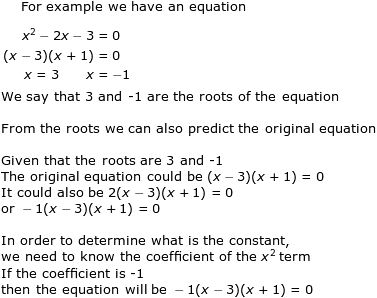### Remainder theorem II

Question 1
The coefficient of theterm in a cubic polynomial f(x) is -1. The roots of f(x) are 1, 2 and k. f(x) when divided by x - 3 gives a remainder of 8.
Find the value of k

First we need to understand what is meant by roots of an equation